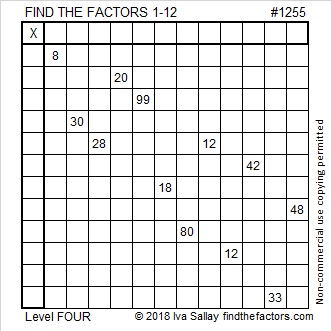# 1255 and Level 4

For this puzzle, you will have to study the twelve clues to figure out where to begin to find your first set of factors. You will then use those factors to figure out the next logical clue to use. Continue the process until all the factors are found. Good luck!Print the puzzles or type the solution in this excel file: 12 factors 1251-1258

Here is some information about the number 1255:

• 1255 is a composite number.
• Prime factorization: 1255 = 5 × 251
• The exponents in the prime factorization are 1 and 1. Adding one to each and multiplying we get (1 + 1)(1 + 1) = 2 × 2 = 4. Therefore 1255 has exactly 4 factors.
• Factors of 1255: 1, 5, 251, 1255
• Factor pairs: 1255 = 1 × 1255 or 5 × 251
• 1255 has no square factors that allow its square root to be simplified. √1255 ≈ 35.425981255 = 251 × 5
Check out those digits on both sides of the equation. Their sameness makes 1255 the18th Friedman number.

1255 is also the hypotenuse of a Pythagorean triple:
753-1004-1255 which is (3-4-5) times 251

This site uses Akismet to reduce spam. Learn how your comment data is processed.